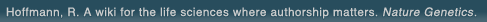# The world's first wiki where authorship really matters (Nature Genetics, 2008). Due credit and reputation for authors. Imagine a global collaborative knowledge base for original thoughts. Search thousands of articles and collaborate with scientists around the globe.

wikigene or wiki gene protein drug chemical gene disease author authorship tracking collaborative publishing evolutionary knowledge reputation system wiki2.0 global collaboration genes proteins drugs chemicals diseases compound
Hoffmann, R. A wiki for the life sciences where authorship matters. Nature Genetics (2008)# Mechanism of electroporative dye uptake by mouse B cells.

The color change of electroporated intact immunoglobulin G receptor (Fc gammaR-) mouse B cells (line IIA1.6) after direct electroporative transfer of the dye SERVA blue G (Mr 854) into the cell interior is shown to be dominantly due to diffusion of the dye after the electric field pulse. Hence the dye transport is described by Fick's first law, where, as a novelty, time-integrated flow coefficients are introduced. The chemical-kinetic analysis uses three different pore states (P) in the reaction cascade ( C <==> P1 <==> P2 <==> P3), to model the sigmoid kinetics of pore formation as well as the biphasic pore resealing. The rate coefficient for pore formation k(p) is dependent on the external electric field strength E and pulse duration tE. At E = 2.1 kV cm(-1) and tE = 200 micros, k(p) = (2.4 +/- 0.2) x 10(3) s(-1) at T = 293 K; the respective (field-dependent) flow coefficient and permeability coefficient are k(f)0 = (1.0 +/- 0.1) x 10(-2) s(-1) and P0 = 2 cm s(-1), respectively. The maximum value of the fractional surface area of the dye-conductive pores is 0.035 +/- 0.003%, and the maximum pore number is Np = (1.5 +/- 0.1) x 10(5) per average cell. The diffusion coefficient for SERVA blue G, D = 10(-6) cm2 s(-1), is slightly smaller than that of free dye diffusion, indicating transient interaction of the dye with the pore lipids during translocation. The mean radii of the three pore states are r(P1) = 0.7 +/- 0.1 nm, r(P2) = 1.0 +/- 0.1 nm, and r(P3) = 1.2 +/- 0.1 nm, respectively. The resealing rate coefficients are k(-2) = (4.0 +/- 0.5) x 10(-2) s(-1) and k(-3) = (4.5 +/- 0.5) x 10)(-3) s(-1), independent of E. At zero field, the equilibrium constant of the pore states (P) relative to closed membrane states ( C) is K(p)0 = [(P)]/[C] = 0.02 +/- 0.002, indicating 2.0 +/- 0.2% water associated with the lipid membrane. Finally, the results of SERVA blue G cell coloring and the new analytical framework may also serve as a guideline for the optimization of the electroporative delivery of drugs that are similar in structure to SERVA blue G, for instance, bleomycin, which has been used successfully in the new discipline of electrochemotherapy.

## References

1. Mechanism of electroporative dye uptake by mouse B cells. Neumann, E., Toensing, K., Kakorin, S., Budde, P., Frey, J. Biophys. J. (1998) [Pubmed]